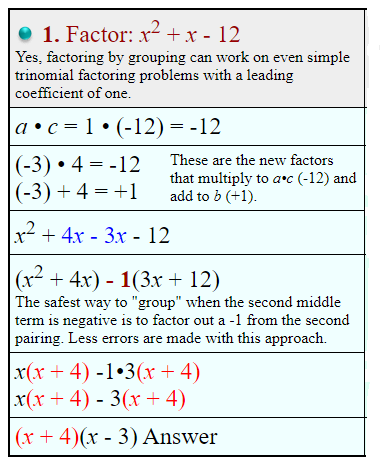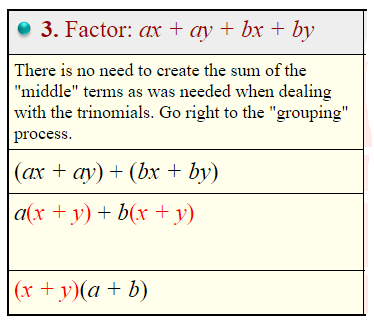## Factoring by Grouping

Factoring by Grouping condensed: x² + bx +c

1. Find the product of 1•c.
2. Find two factors of c that add to up to b.
3. Split the middle term into two terms using these factors.
4. Group the four terms to form two pairs.
5. Factor each pair.
6. Factor out the common (shared) binomial parenthesis.

There are many different applications of factoring by grouping.
We will be examining factoring by grouping as it applies to trinomials of the form ax2 + bx + c.
Notice:(x + 4)(x + 6) = x2 + 4x + 6x + 24 = x2 + 10x + 24

Process of Factoring by Grouping:To get the “factoring by grouping” process started, you are looking for two numbers that multiply to ac, and whose sum is equal to b. These two numbers will help form the new “middle terms”, which will allow for grouping and factoring to arrive at the answer.

Example:Example:It was stated that multiplying binomials often creates two middle terms that are “like” terms. But, of course, not ALL binomial products produce “like” middle terms.
Consider: (a + b)(x + y) = ax + ay + bx + by
In this expression, there is no factor (other than 1) that is common to all four terms. There is, however, a common factor of a for the first two terms, and a common factor of b for the last two terms.
So, “factoring by grouping” will be a strategy for dealing with finding the factors of an expression such as
ax + ay + bx + by.Example: